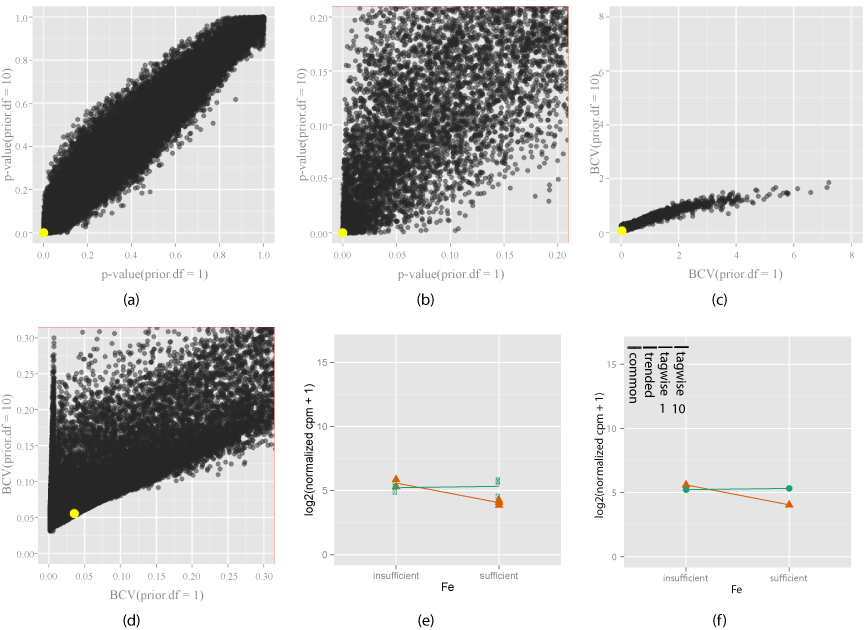Figure 7: Exploring how the shrinkage parameter (prior.df) choice a_ects the di_erential expression of EV (orange) when gene signi_cantly di_erentially expressed with both prior.df = 1 and 10. Two di_erent shrinkage values(prior.df = 1 and prior.df = 10) are chosen, and compared using the change in p- values (7(a)) and zoomed view (7(b)), BCV (7(c)) and zoomed view (7(d)). Yellow indicates gene under investigation, chosen by mouse action on the plot. The interaction plot (7(e)) for this gene, and the model estimates are shown (7(f)). Bars in the model estimates plot (7(f)) show the changes in di_erent dispersion estimates, for tagwise estimates, number means prior.df. Signi_cance of this gene is e_ectively unchanged by shrinkage, as seen that the p-value is similar in value for both shrinkage values, and observed dispersion is small for both RPA (green) and EV (orange) (7(e)). So this is a gene that is not a_ected much by the choice of shrinkage.